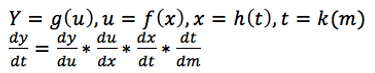### Differentiation Rules

#### Chain Rule

###### Where is it used?

The chain rule is used where the required for differentiation of equations that have layers to them. For example, finding dy/dx in y=f(u), where u=g(x).

###### What is the Formula?

Where g(u) is the first layer and f(x) is the second layer and the required differentiation that needs to be found is dy/dx.

The chain rule works with an infinite number of layers. Of course, the more layers there are the more difficult it becomes. For example, the formula for the chain rule for four layers would be.

Step 2: Substitute  g'(u) and f'(x) into the chain rule formula.

###### Test Style Chain Rule Questions

Download a free worksheet containing 5 chain rule questions with worked solutions and allocated marks for each question.Step 3: Substitute other variables until there is only one variable. In this example, we will substitute u with 2x^2+5x so the equation only has one variable.

Step 4: Expand and then Simplify the equation.###### Worked Example

In this example, we will differentiate (dy/dx) y=5u^2+6u+3, u=2x^2+5x

Step 1: Determine the equations g'(x), f(x)